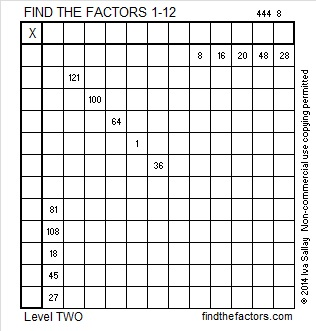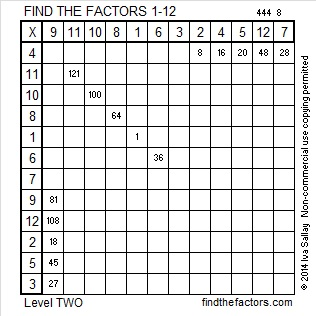# 285 and Level 2

• 285 is a composite number.
• Prime factorization: 285 = 3 x 5 x 19
• The exponents in the prime factorization are 1, 1, and 1. Adding one to each and multiplying we get (1 + 1)(1 + 1)(1 + 1) = 2 x 2 x 2 = 8. Therefore 285 has 8 factors.
• Factors of 285: 1, 3, 5, 15, 19, 57, 95, 285
• Factor pairs: 1 x 185, 3 x 95, 5 x 57, or 15 x 19
• 285 has no square factors that allow its square root to be simplified. √285 ≈ 16.882Print the puzzles or type the factors on this excel file: 12 Factors 2014-11-03This site uses Akismet to reduce spam. Learn how your comment data is processed.